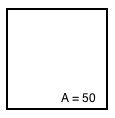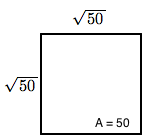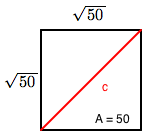### Home > INT2 > Chapter 7 > Lesson 7.1.3 > Problem7-44

7-44.

How long is the longest line segment that will fit inside a square of area $50$ square units? Show all work.

Draw a diagram. A square labeled, A, = 50.

Area of a square = s², where s = length of a side. Side labels added to square, on top & left sides, square root of 50.

${s}=\sqrt{50}$

Use the Pythagorean Theorem to solve for $c$.  Added to square, diagonal from, bottom left to top right, labeled, c.